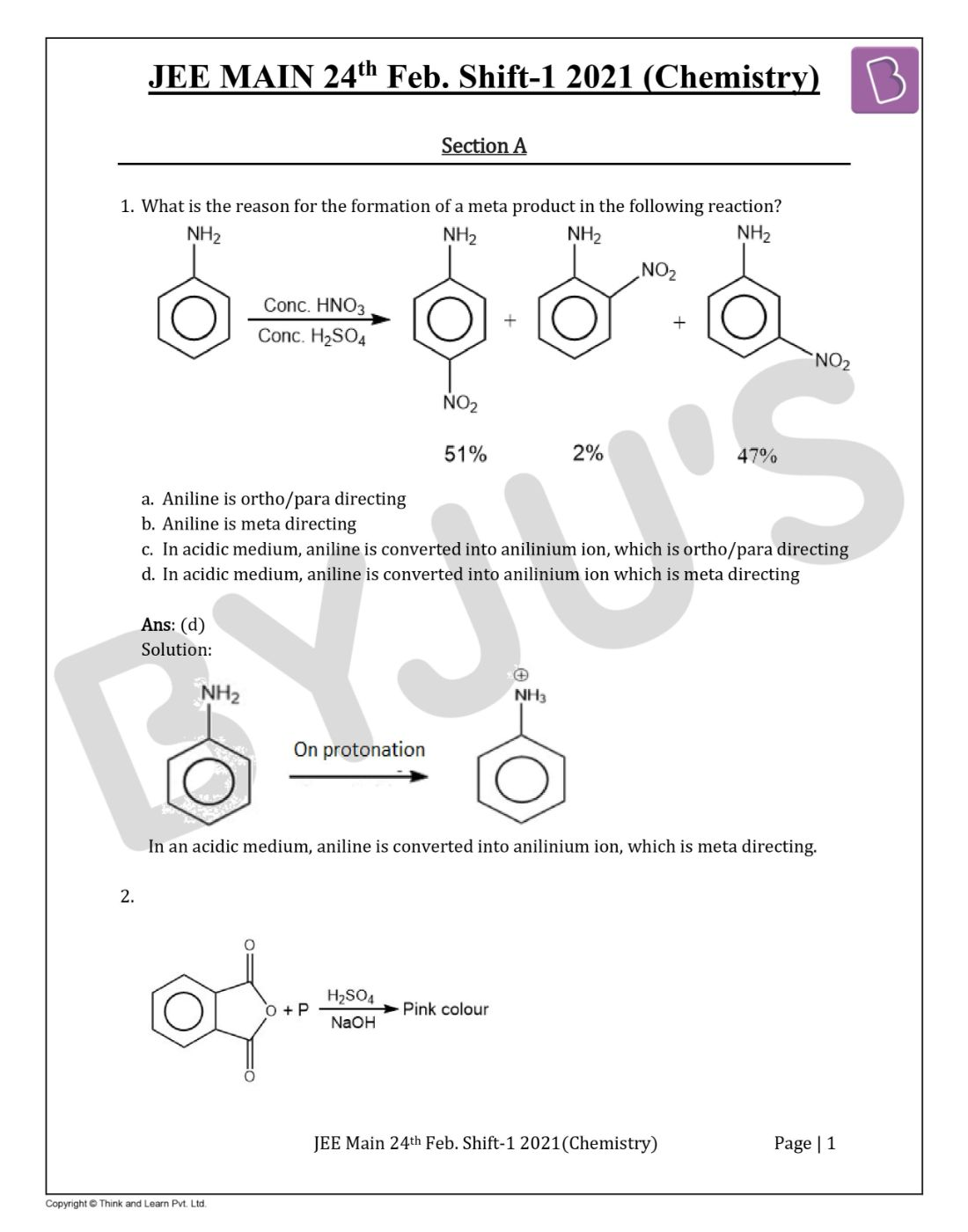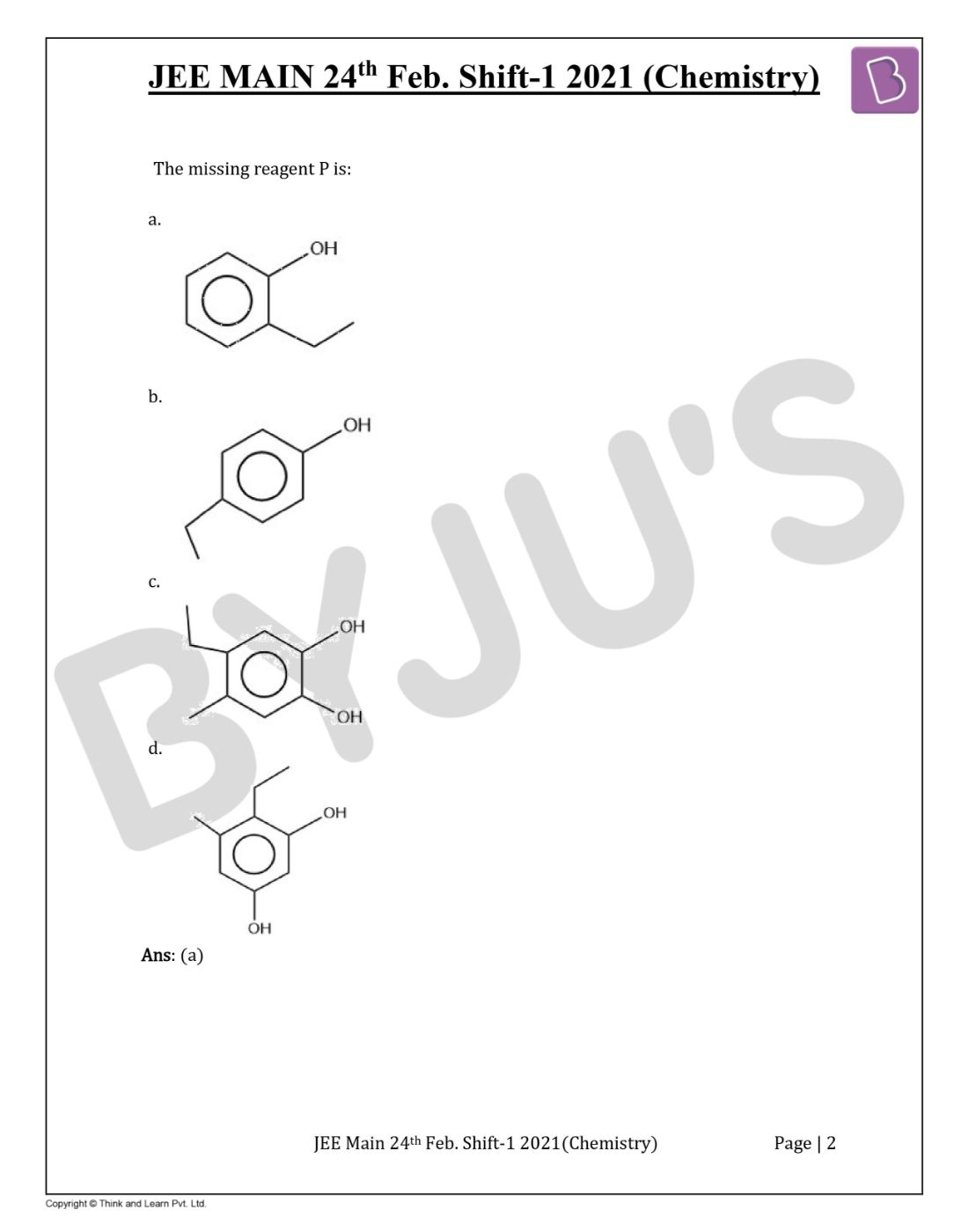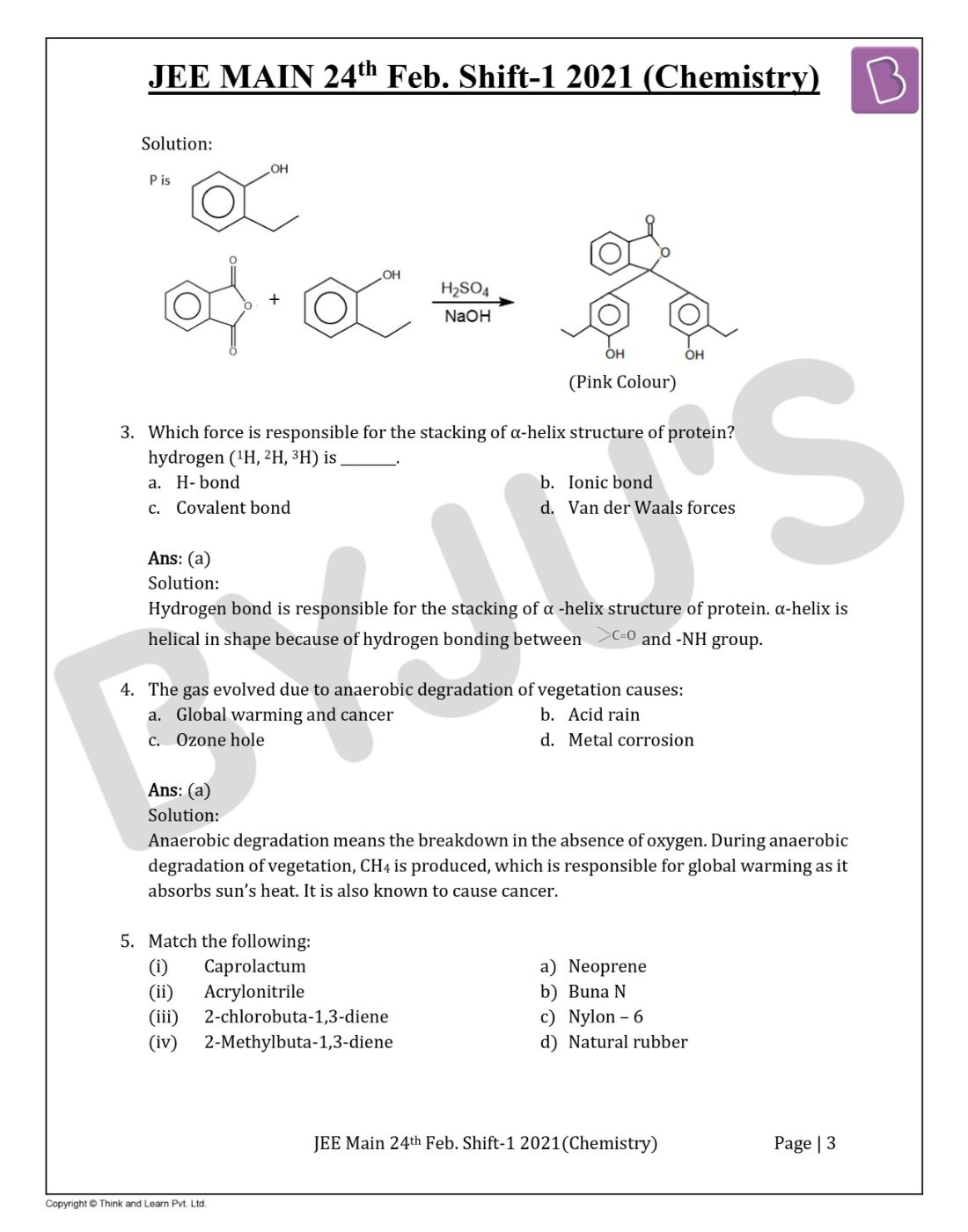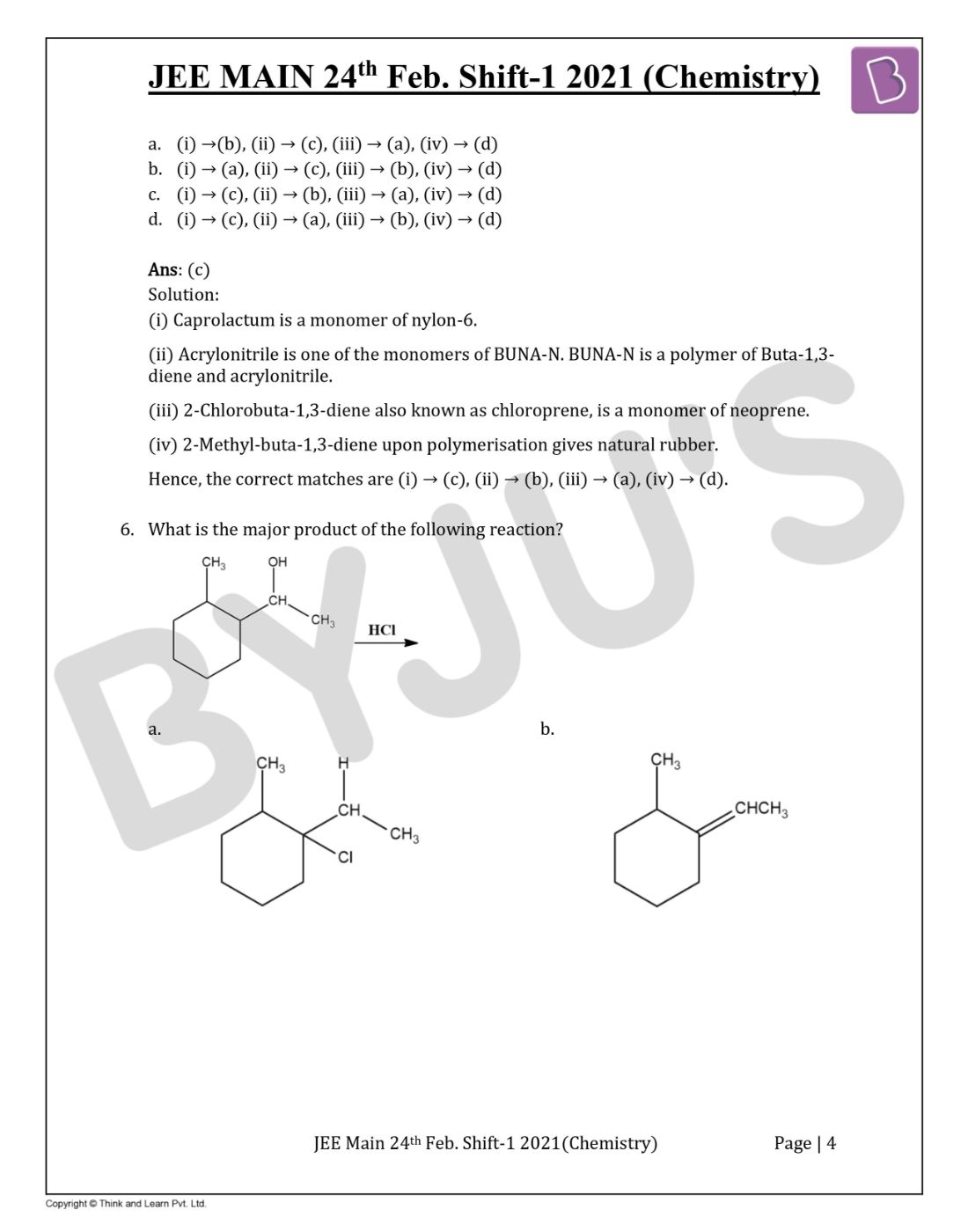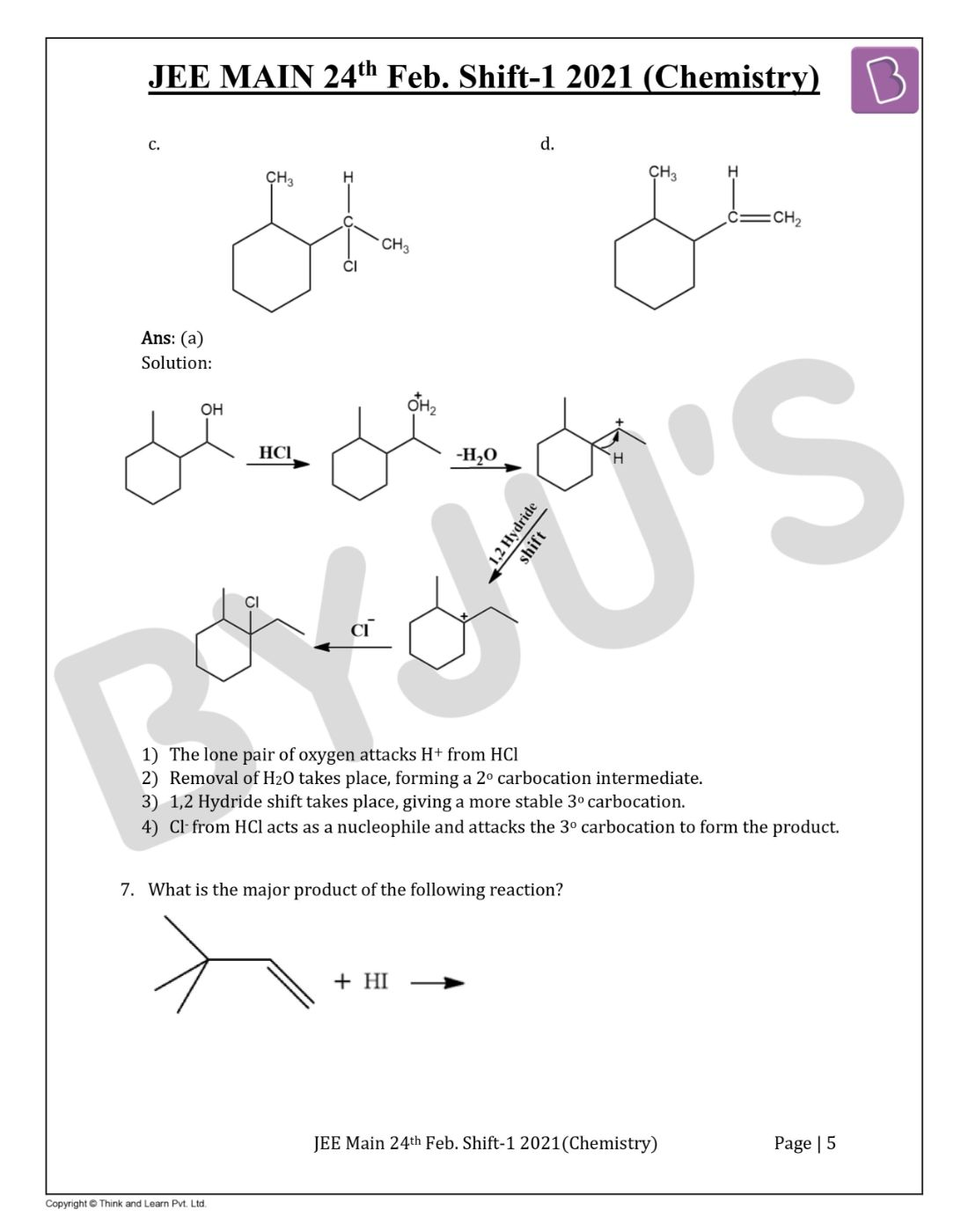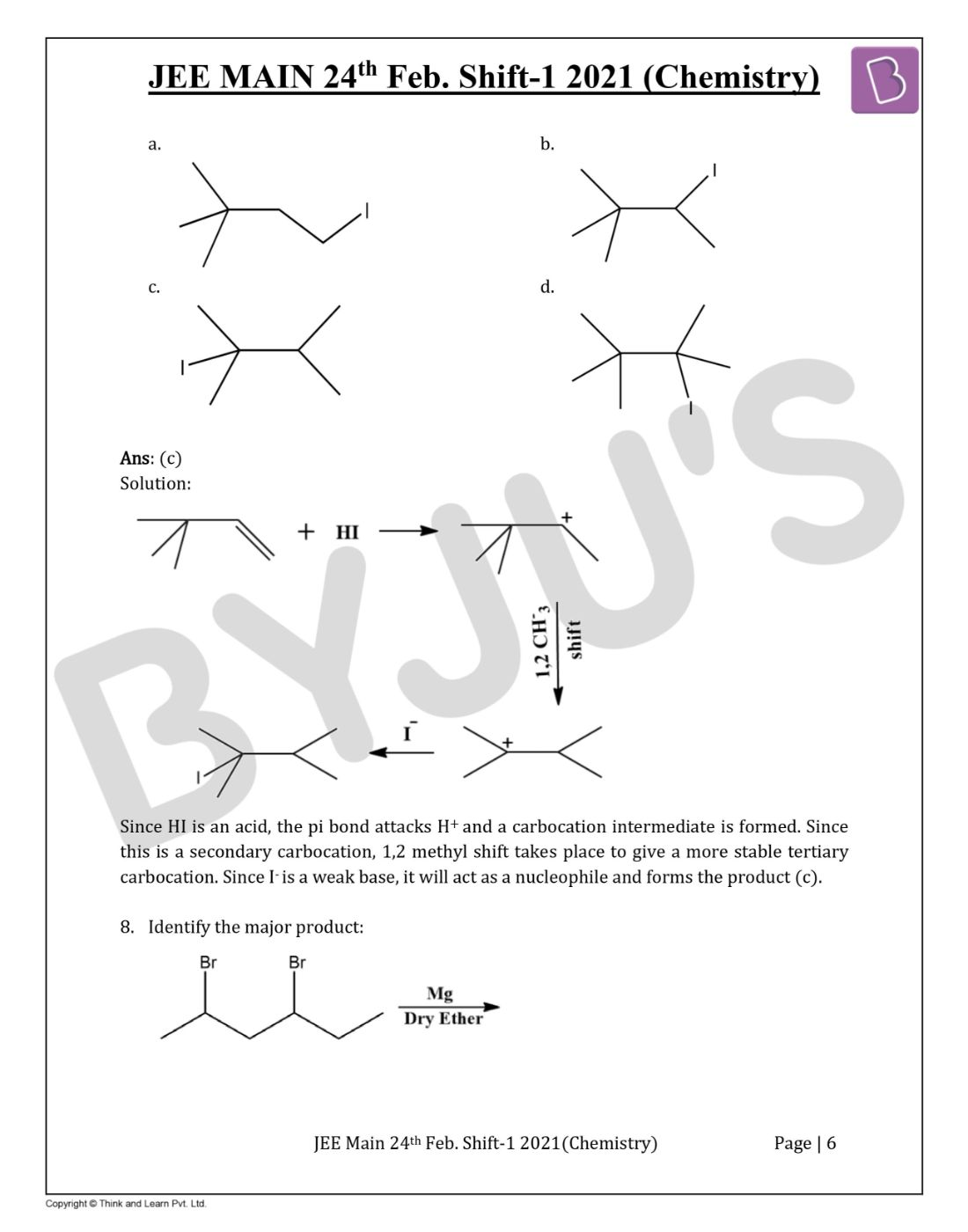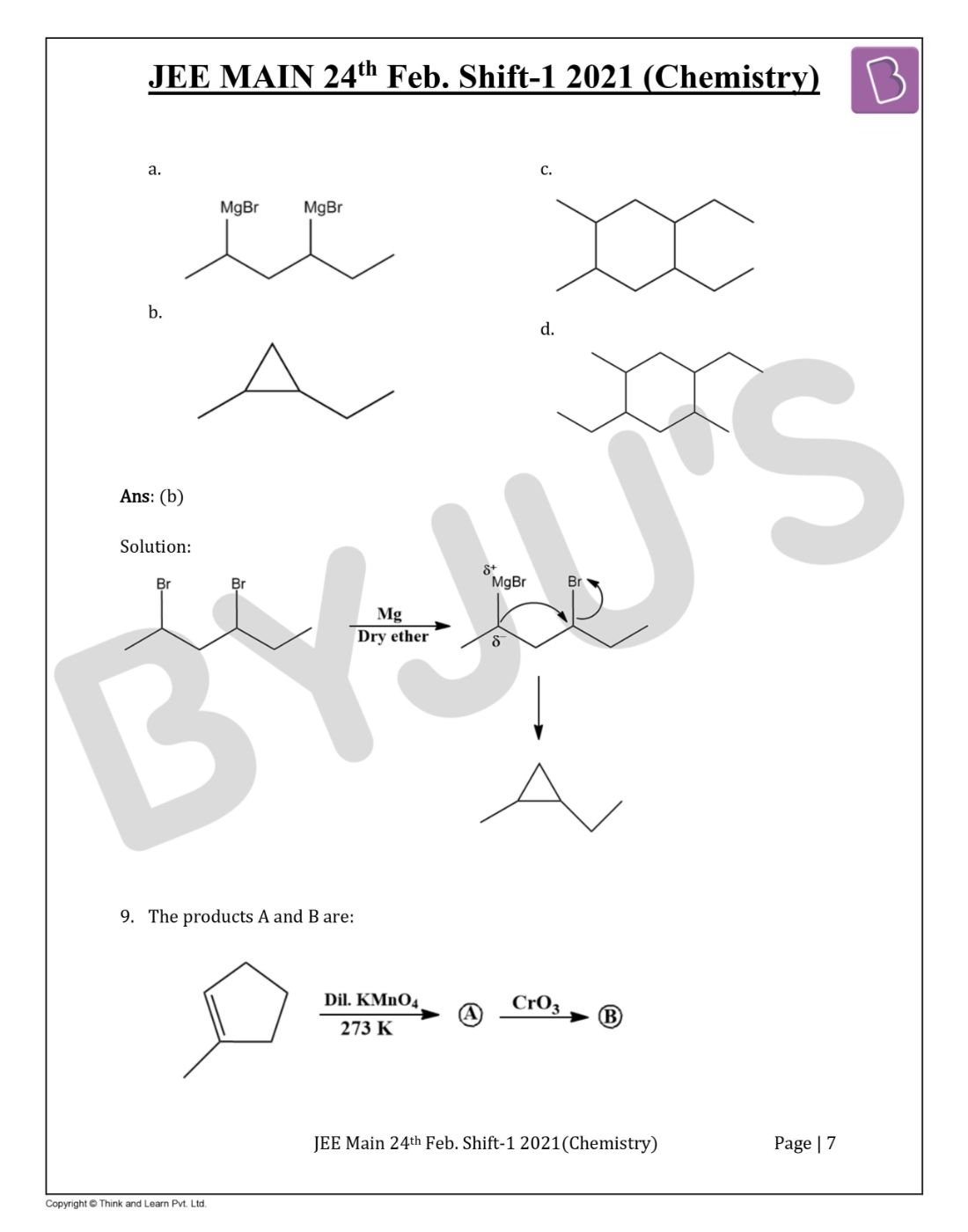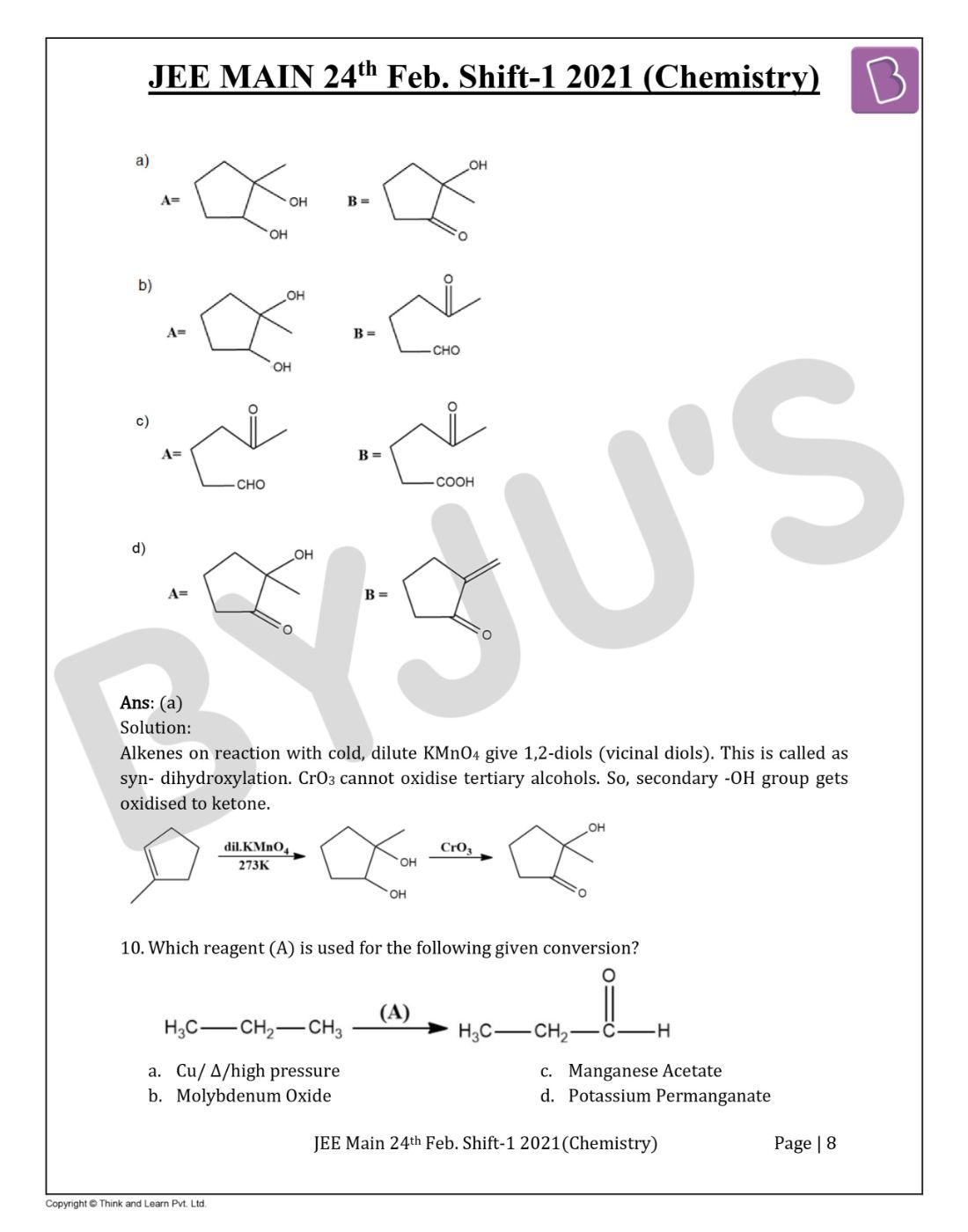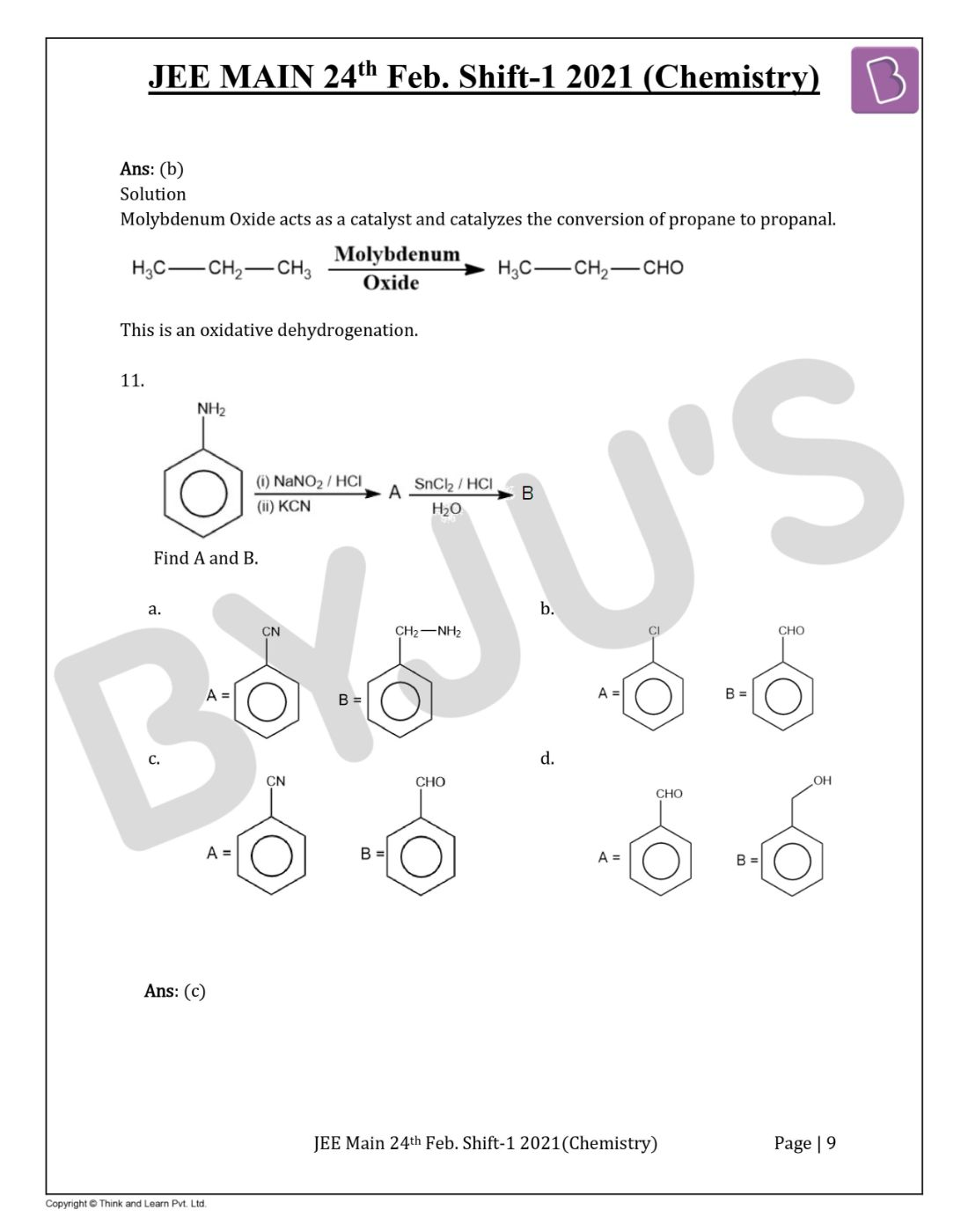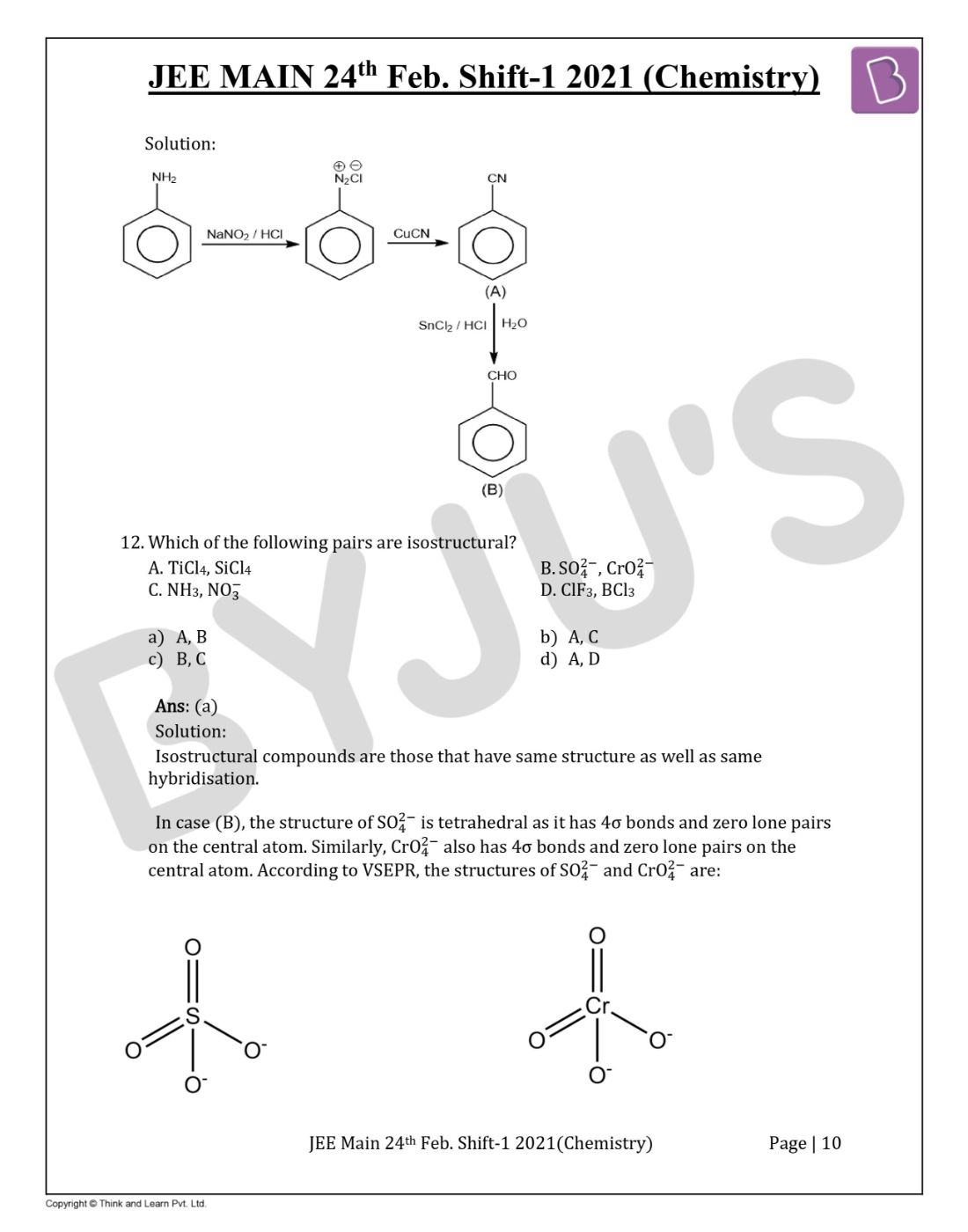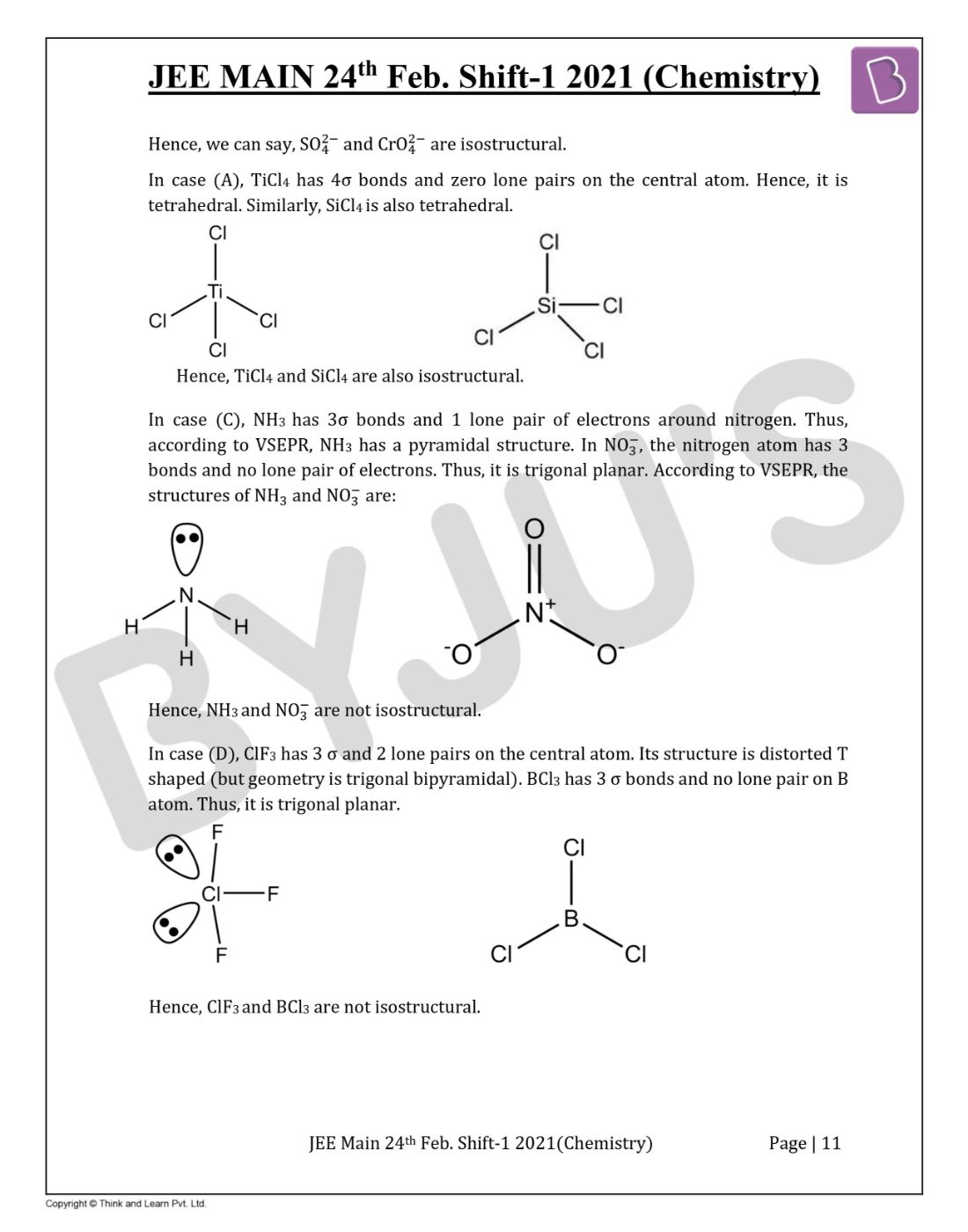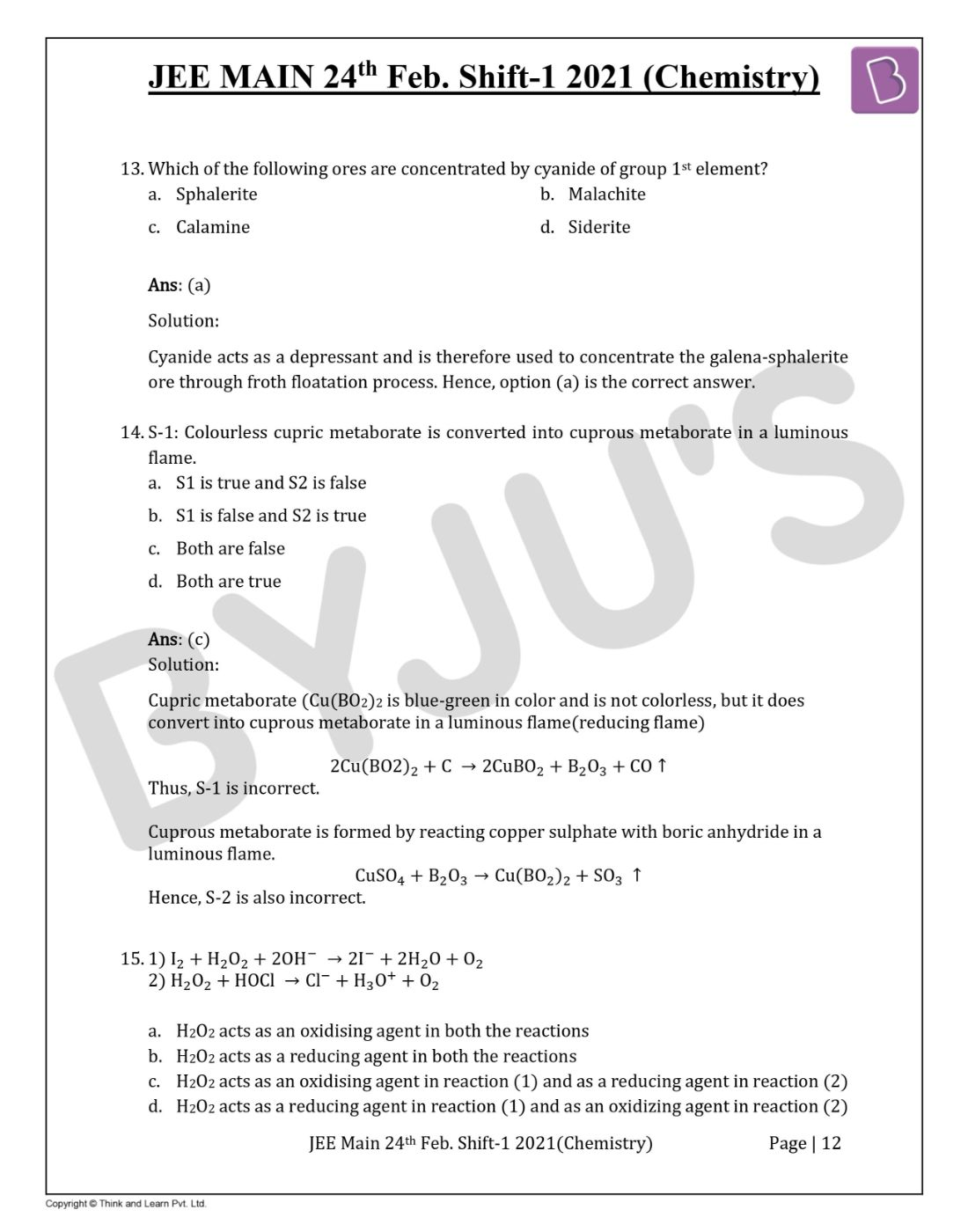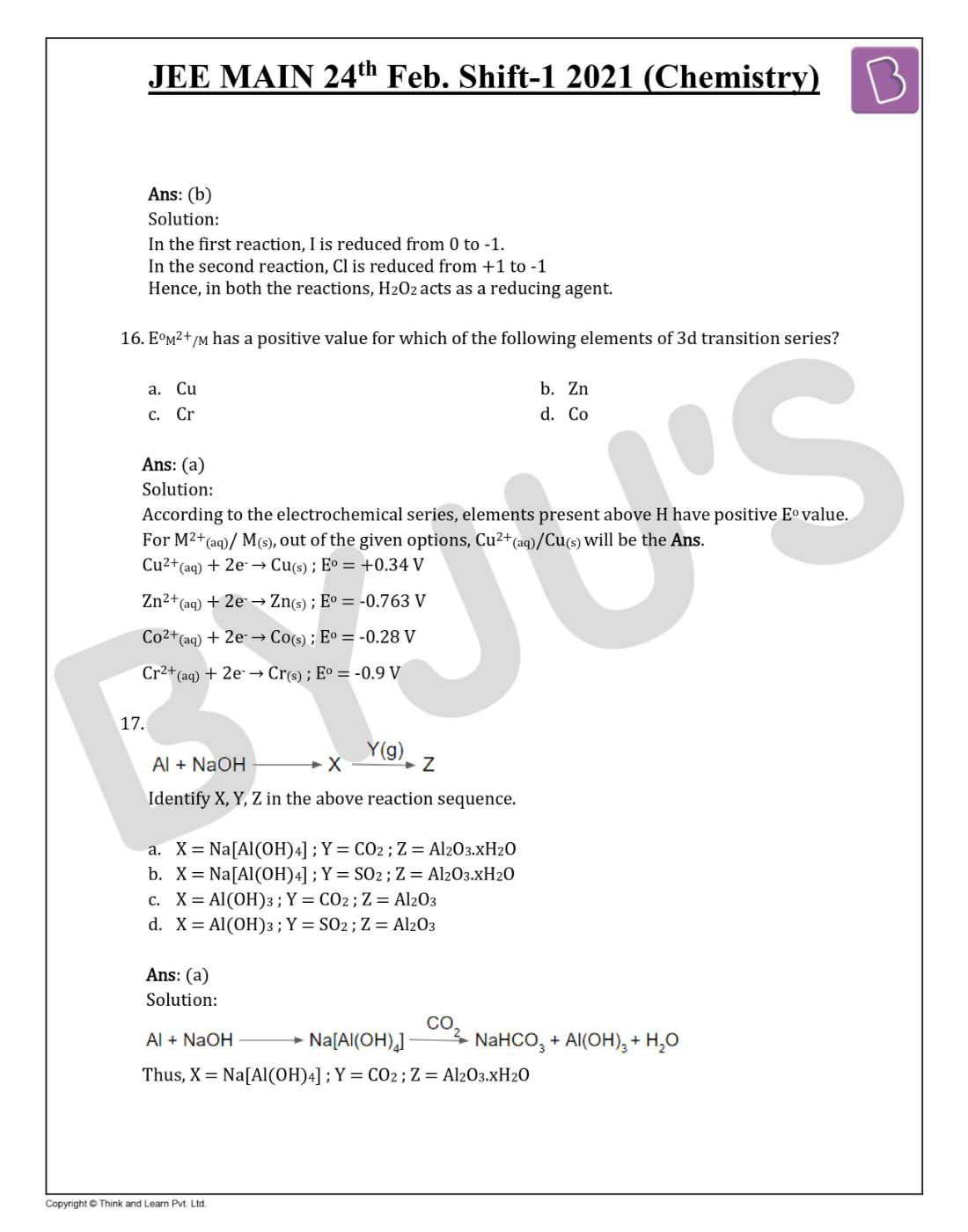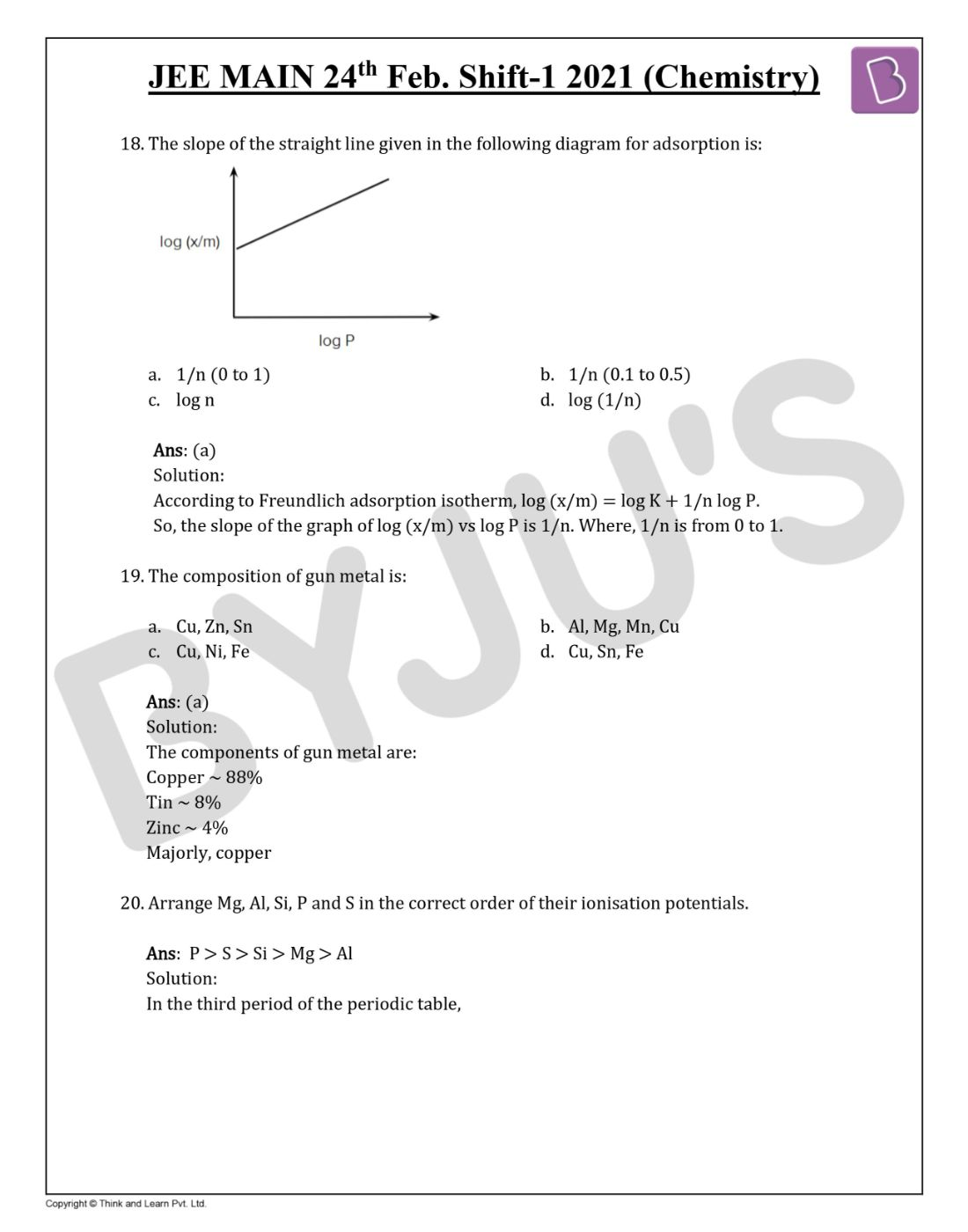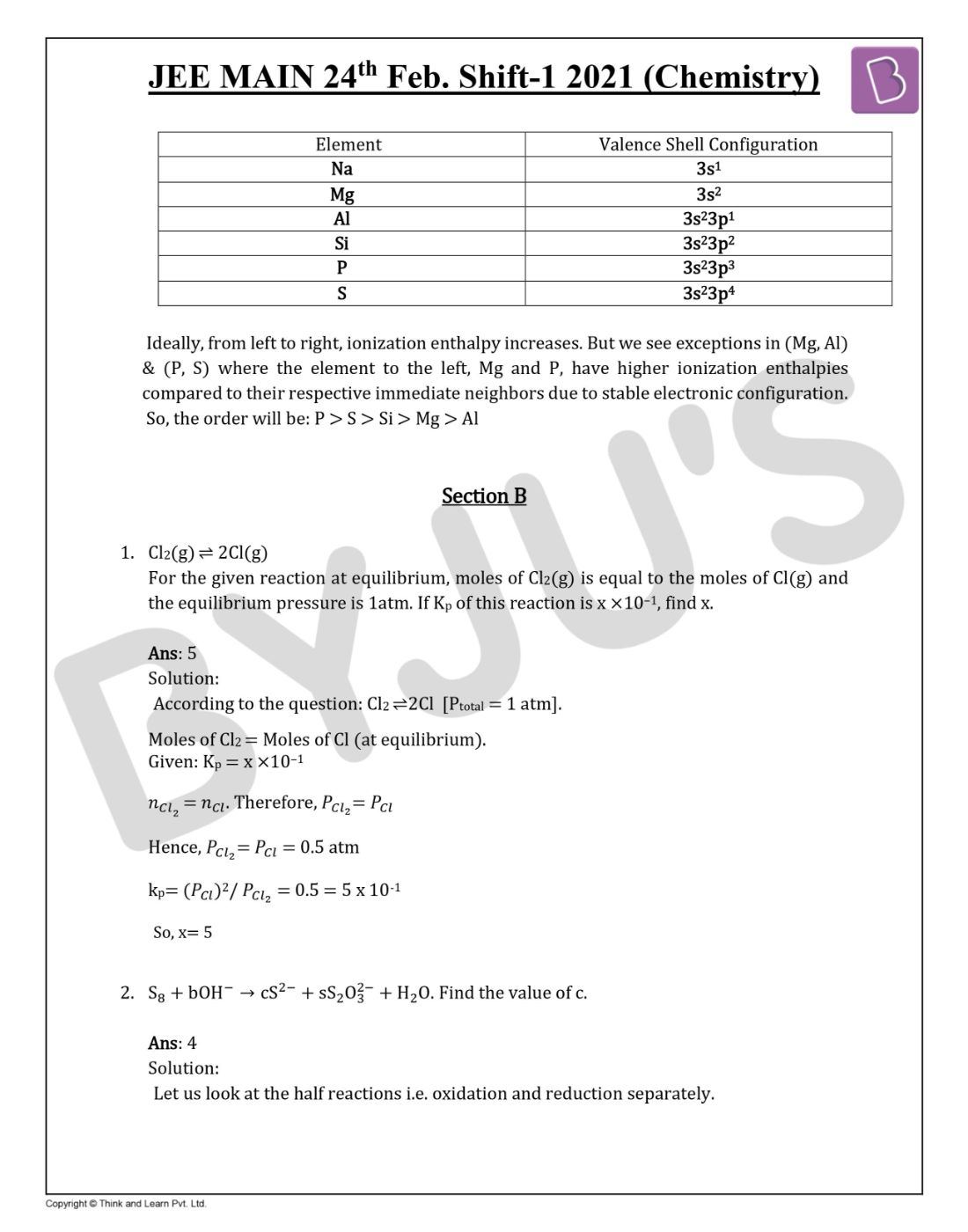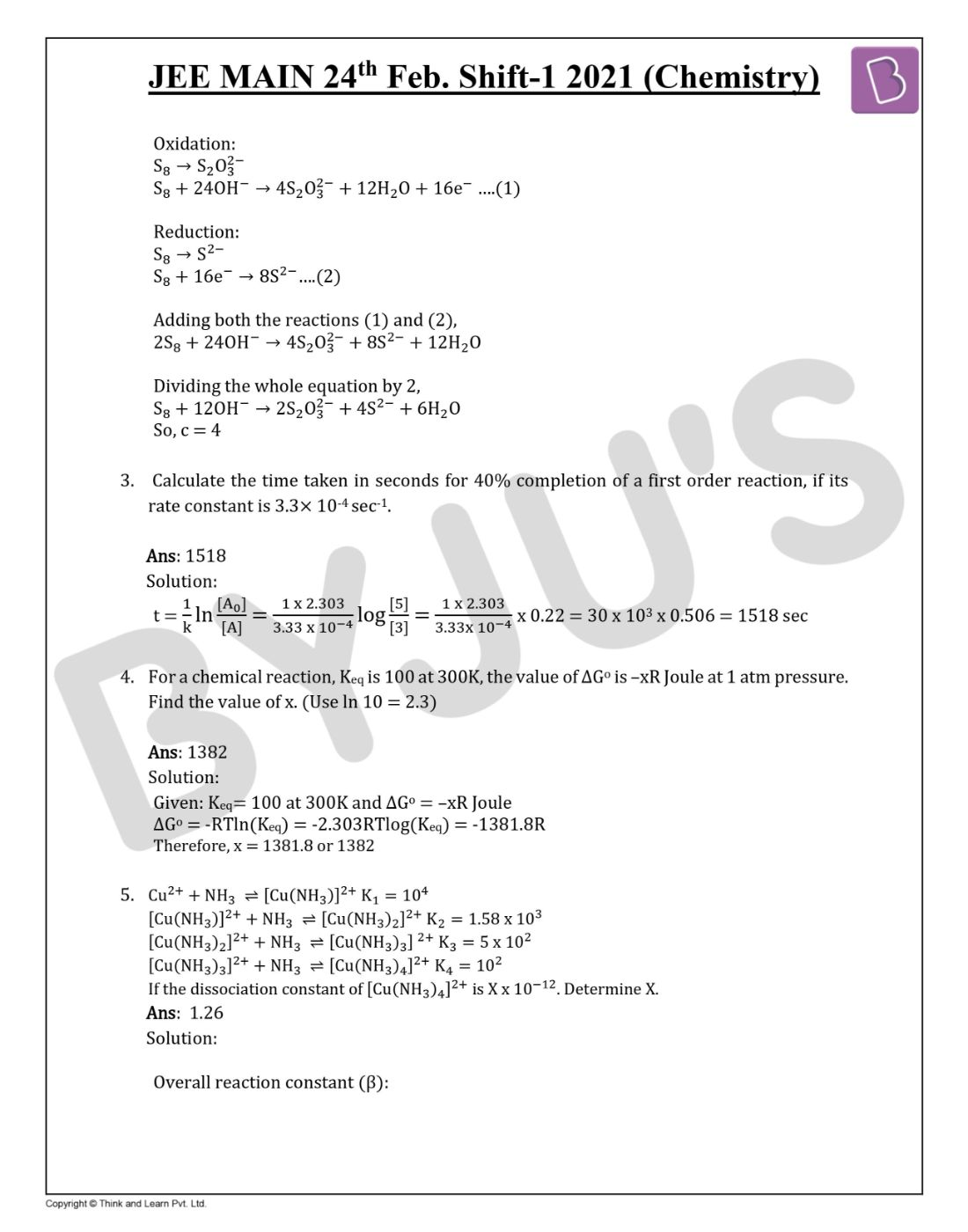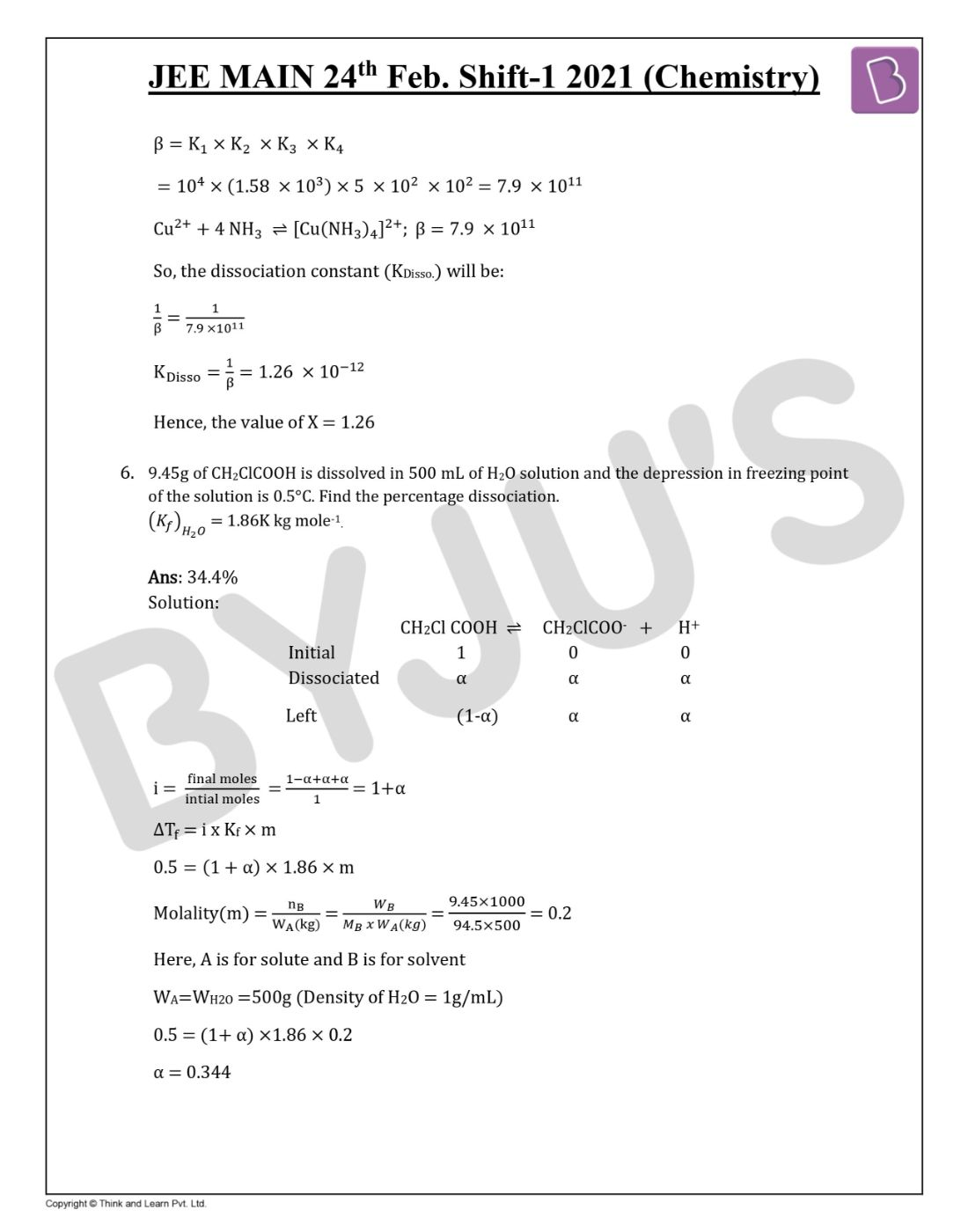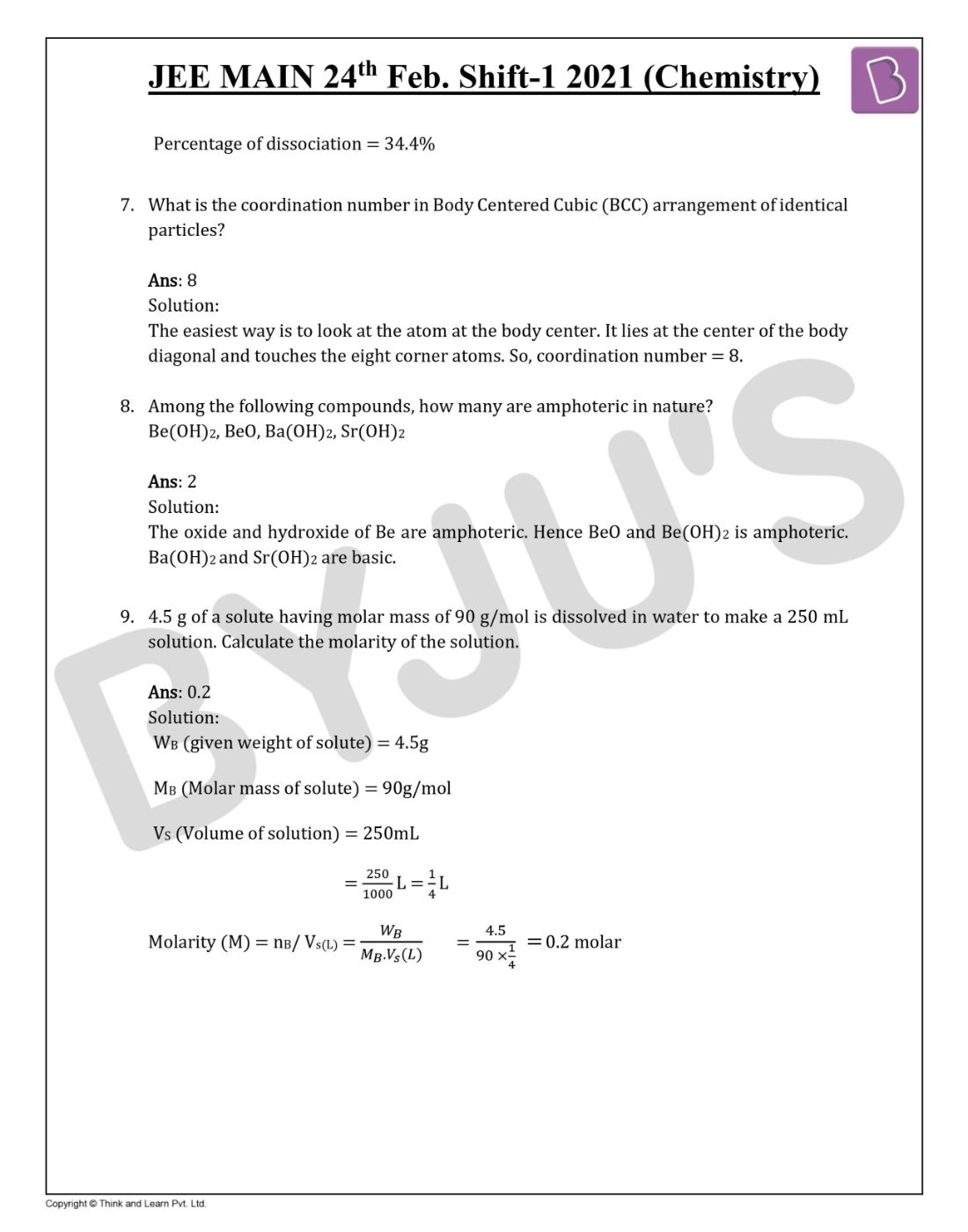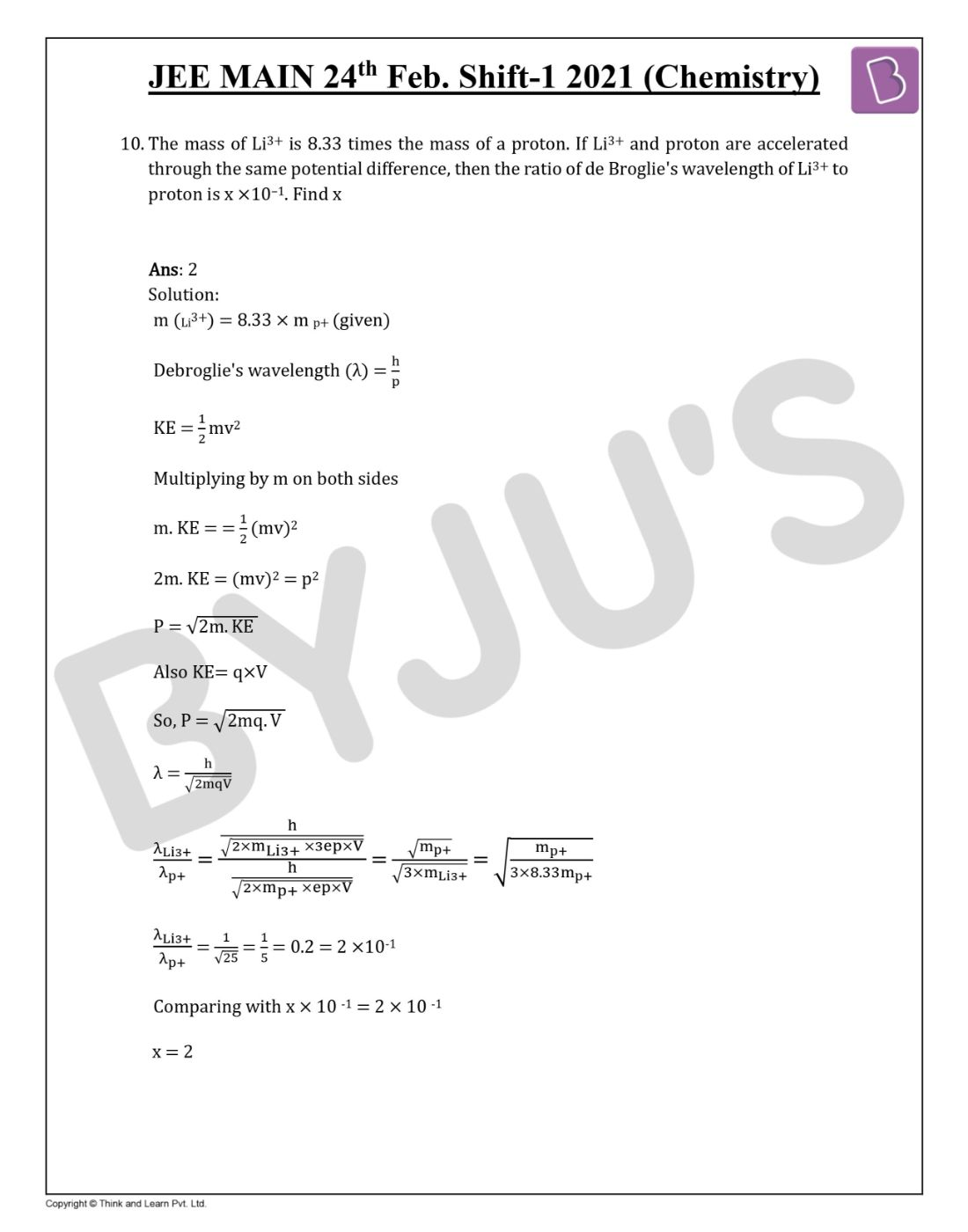Win up to 100% scholarship on Aakash BYJU'S JEE/NEET courses with ABNAT Win up to 100% scholarship on Aakash BYJU'S JEE/NEET courses with ABNAT

# JEE Main 2021 Question Paper Chemistry Feb 24 Shift-1

JEE Main 2021 Chemistry question paper with solutions for Feb 24 session shift 1 is updated here to assist students in learning the correct answers to each of the problems discussed in the paper. Each question present in JEE Main 2021 Chemistry paper has been thoughtfully answered by our experts and students can further have an effective revision of the important topics

Additionally, as students view the JEE Main 2021 Chemistry question paper and go through the solutions they will get an overall nature of the questions including difficulty level, types or format and the marks weightage of each question. Students can directly view the questions and solutions on this page or they can also download the question paper PDF and use it at a later stage during their study hours.

Feb 24 Shift 1 – Chemistry Question Paper and Solutions

Question 1. What is the reason for the formation of a meta product in the following reaction?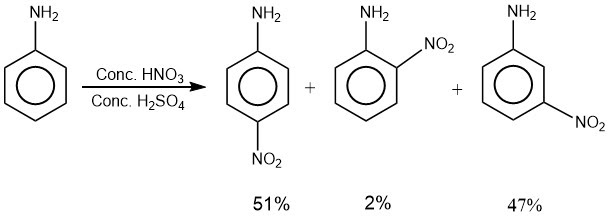a. Aniline is ortho/para directing
b. Aniline is meta directing
c. In acidic medium, aniline is converted into anilinium ion, which is ortho/para directing
d. In acidic medium, aniline is converted into anilinium ion which is meta directing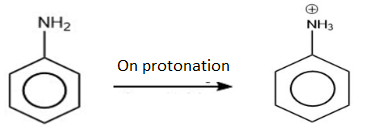In an acidic medium, aniline is converted into anilinium ion, which is meta directing.

Question 2. The missing reagent P is: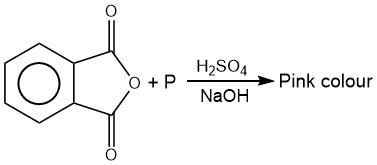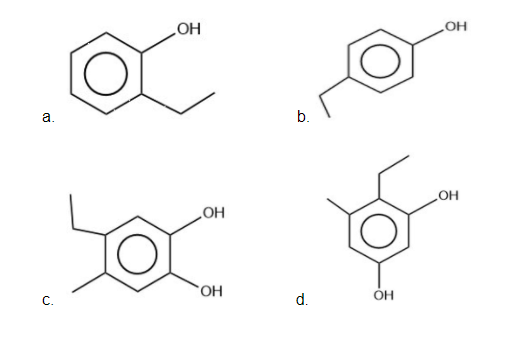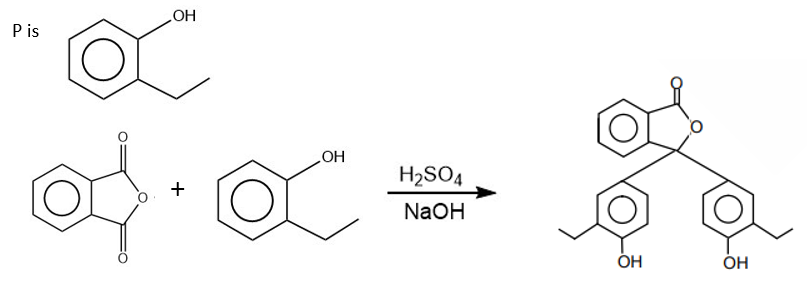(Pink Colour)

Question 3. Which force is responsible for the stacking of the a-helix structure of protein? Hydrogen (1H, 2H, 3H) is ________

a. H-bond
b. Ionic bond
c. Covalent bond
d. Van der Waals forces

Hydrogen bond is responsible for the stacking of a-helix structure of protein. a-helix is helical in shape because of hydrogen bonding between >C=O and -NH group.

Question 4. The gas evolved due to anaerobic degradation of vegetation causes:

a. Global warming and cancer
b. Acid rain
c. Ozone hole
d. Metal corrosion

Anaerobic degradation means the breakdown in the absence of oxygen. During anaerobic degradation of vegetation, CH4 is produced, which is responsible for global warming as it absorbs sun’s heat. It is also known to cause cancer.

Question 5. Match the following:

(i) Caprolactam

(ii) Acrylonitrile

(iii) 2-chlorobuta-1,3-diene

(iv) 2-Methylbuta-1,3-diene

p>(a) Neoprene

(b) Buna N

(c) Nylon – 6

(d) Natural rubber

a. (i) ?(b), (ii) ? (c), (iii) ? (a), (iv) ? (d)
b. (i) ? (a), (ii) ? (c), (iii) ? (b), (iv) ? (d)
c. (i) ? (c), (ii) ? (b), (iii) ? (a), (iv) ? (d)
d. (i) ? (c), (ii) ? (a), (iii) ? (b), (iv) ? (d)

(i) Caprolactum is a monomer of nylon-6.

(ii) Acrylonitrile is one of the monomers of BUNA-N. BUNA-N is a polymer of Buta-1,3-diene and acrylonitrile.

(iii) 2-Chlorobuta-1,3-diene also known as chloroprene, is a monomer of neoprene.

(iv) 2-Methyl-buta-1,3-diene upon polymerisation gives natural rubber.

Hence, the correct matches are (i) ? (c), (ii) ? (b), (iii) ? (a), (iv) ? (d).

Question 6. What is the major product of the following reaction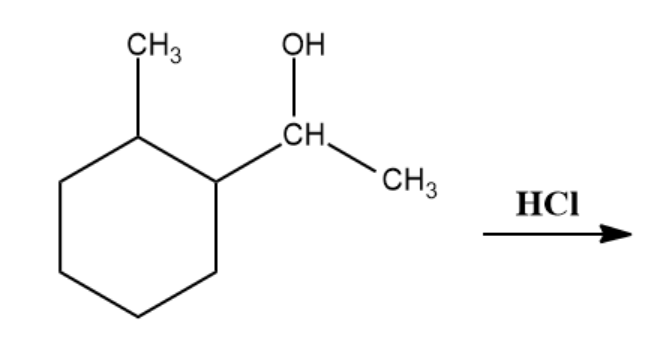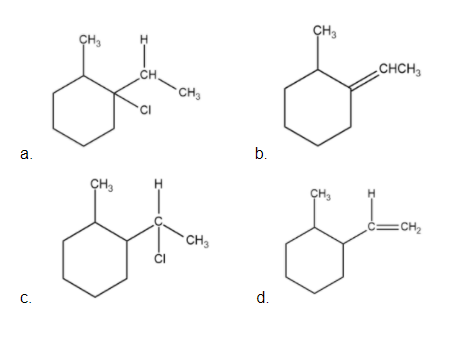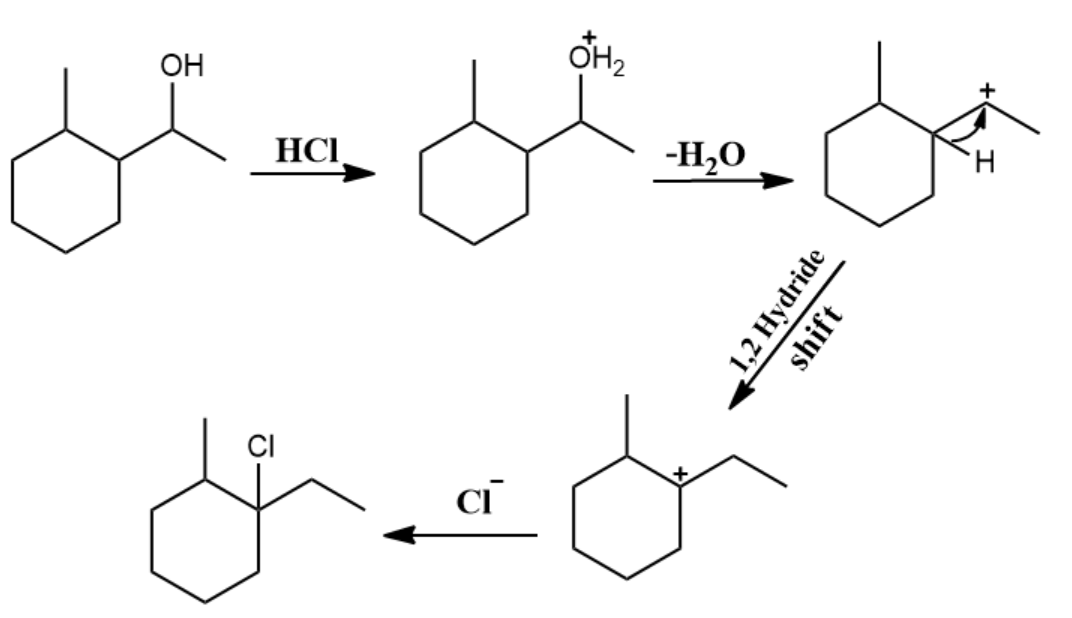1. The lone pair of oxygen attacks H+ from HCl

2. Removal of H2O takes place, forming a 2o carbocation intermediate.

3. 1,2 Hydride shift takes place, giving a more stable 3o carbocation.

4. Clfrom HCl acts as a nucleophile and attacks the 3o carbocation to form the product.

Question 7. What is the major product of the following reaction?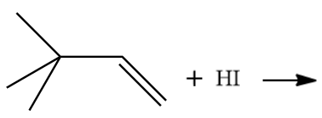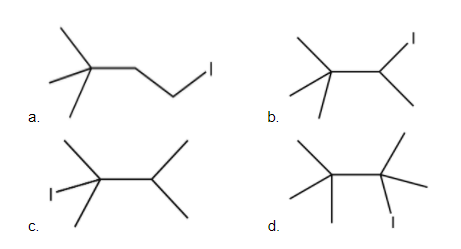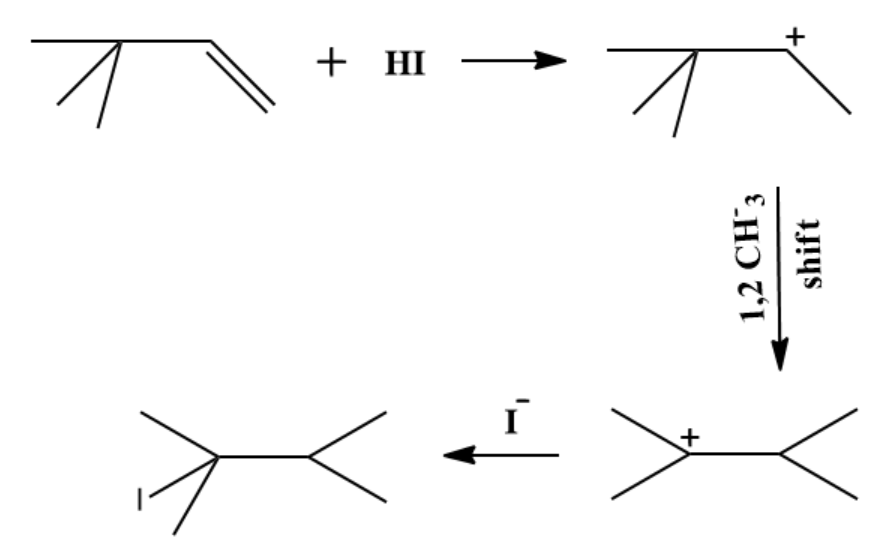Since HI is an acid, the pi bond attacks H+ and a carbocation intermediate is formed. Since this is a secondary carbocation, 1,2 methyl shift takes place to give a more stable tertiary carbocation. Since Iis a weak base, it will act as a nucleophile and forms the product (c).

Question 8. Identify the major product: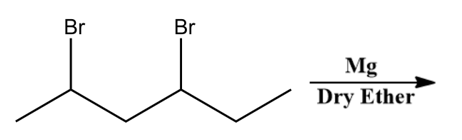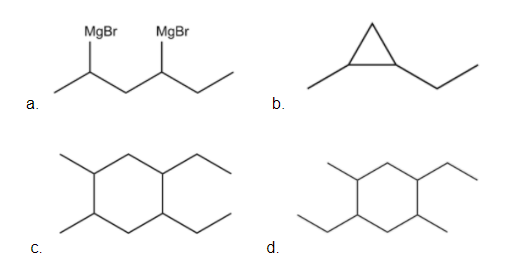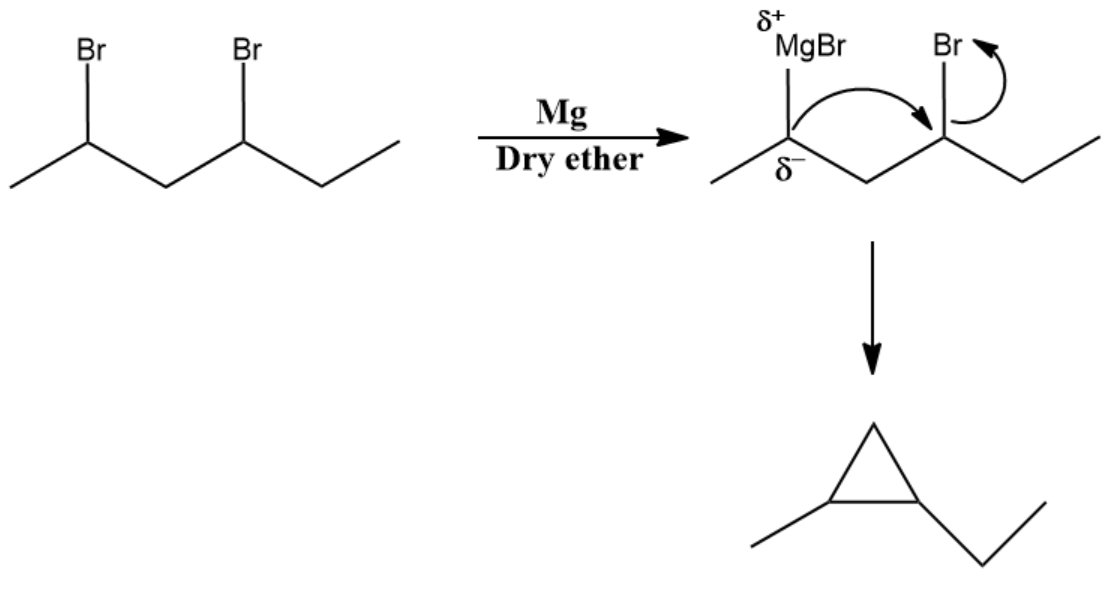Question 9. The products A and B are: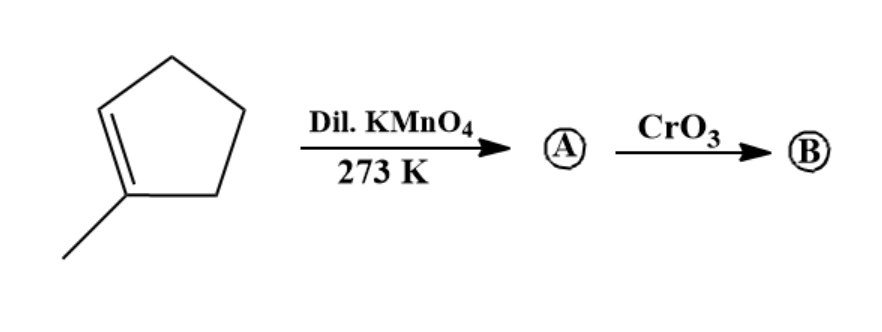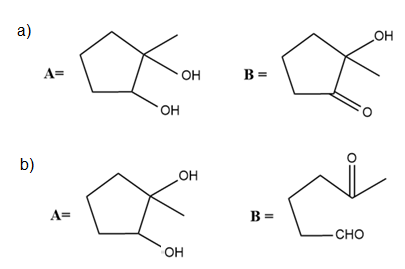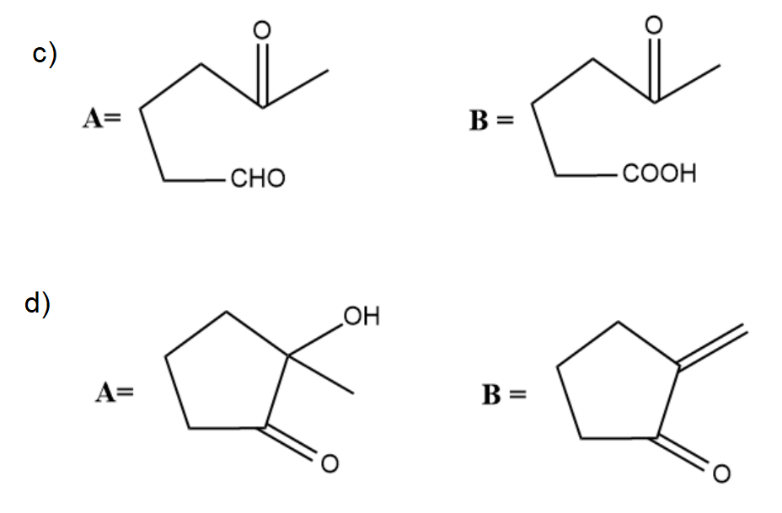Alkenes reacting with cold, dilute KMnO4 will give 1,2-diols (vicinal diols). This is called syn- dihydroxylation. CrO3 cannot oxidise tertiary alcohols. So, the secondary -OH group gets oxidised to ketone.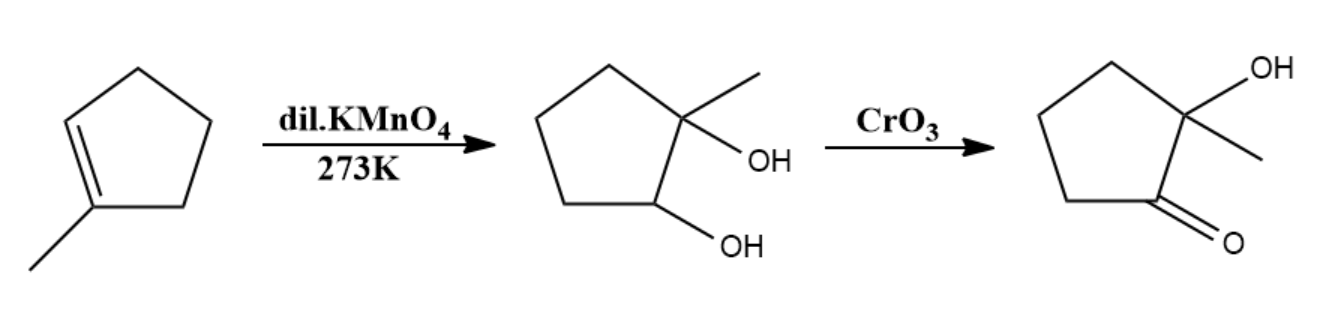Question 10. Which reagent (A) is used for the following given conversion?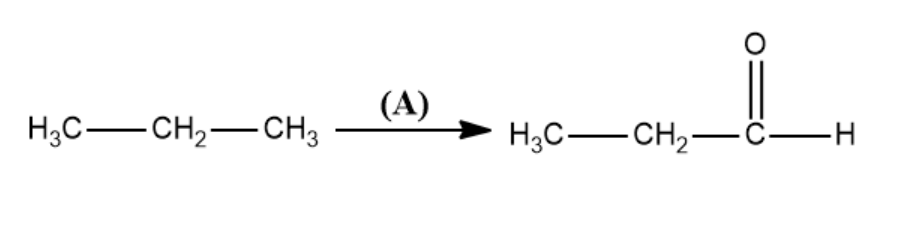a. Cu / ? / high pressure
b. Molybdenum Oxide
c. Manganese Acetate
d. Potassium Permanganate

Molybdenum Oxide acts as a catalyst and catalyzes the conversion of propane to propanal.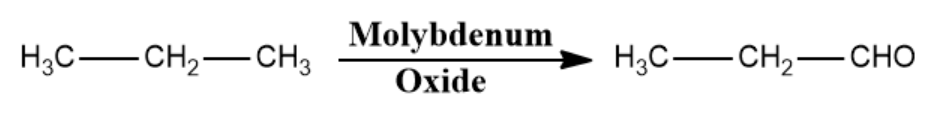This is an oxidative dehydrogenation.

Question 11. Find A and B.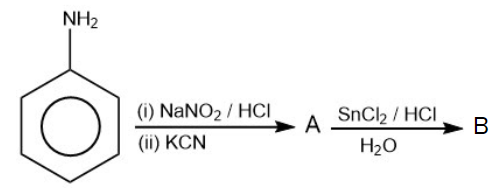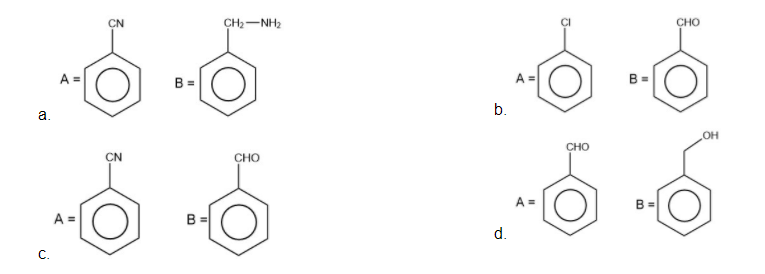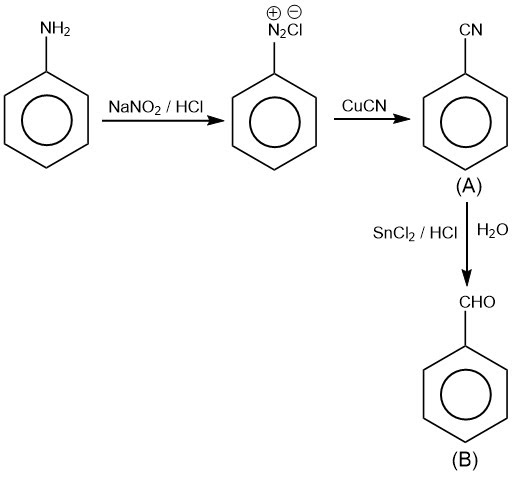Question 12. Which of the following pairs are isostructural

a) TiCl4, SiCl4

b) SO2-3, CrO2-3

c) NH3, NO3

d) ClF3, BCl3

a. A, B
b. A, C
c. B, C
d. A, D

Isostructural compounds are those that have the same structure as well as same hybridisation.

In case (B), the structure of SO2-4 is tetrahedral as it has 4s bonds and zero lone pairs on the central atom. Similarly, CrO2-4 also has 4s bonds and zero lone pairs on the central atom. According to VSEPR, the structures of SO2-4 and CrO2-4 are: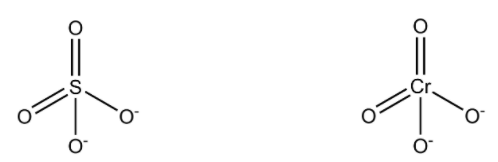Hence, we can say, SO2-4 and CrO2-4 are isostructural.

In case (A), TiCl4 has 4s bonds and zero lone pairs on the central atom. Hence, it is tetrahedral. Similarly, SiCl4 is also tetrahedral.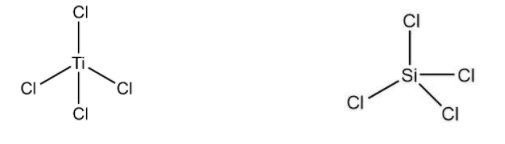Hence, TiCl4 and SiCl4 are also isostructural.

In case (C), NH3 has 3s bonds and 1 lone pair of electrons around nitrogen. Thus, according to VSEPR, NH3 has a pyramidal structure. In NO3, the nitrogen atom has 3 bonds and no lone pair of electrons. Thus, it is trigonal planar. According to VSEPR, the structures of NH3 and NO3 are: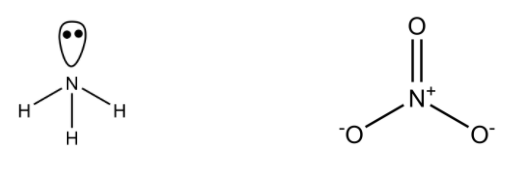Hence, NH3 and NO3 are not isostructural.

In case (D), ClF3 has 3 s and 2 lone pairs on the central atom. Its structure is distorted T shaped (but geometry is trigonal bipyramidal). BCl3 has 3 s bonds and no lone pair on the B atom. Thus, it is trigonal planar.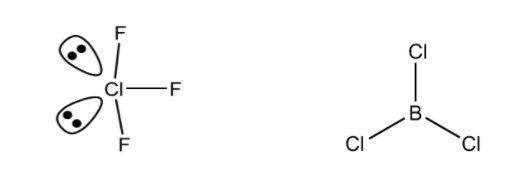Hence, ClF3 and BCl3 are not isostructural.

Question 13. Which of the following ores are concentrated by cyanide of group 1st element?

a. Sphalerite
b. Malachite
c. Calamine
d. Siderite

Cyanide acts as a depressant and is therefore used to concentrate the galena-sphalerite ore through the froth floatation process. Hence, option (a) is the correct answer.

Question 14. S-1: Colourless cupric metaborate is converted into cuprous metaborate in a luminous flame.

a. S-1 is true and S-2 is false
b. S-1 is false and S-2 is true
c. Both are false
d. Both are true

Cupric metaborate (Cu(BO2)2 is blue-green in color and is not colorless, but it does convert into cuprous metaborate in a luminous flame(reducing flame)

2Cu(BO2)2 + C ? 2CuBO2 + B2O3 + CO?

Thus, S-1 is incorrect.

Cuprous metaborate is formed by reacting copper sulphate with boric anhydride in a luminous flame.

CuSO4 + B2O3 ? Cu(BO2)2 + SO3?

Hence, S-2 is also incorrect.

Question 15. In the given reactions,

1) I2 + H2O2 + 2OH ? 2I + 2H2O + O2

2) H2O2 + HOCl ? Cl + H3O+ + O2

a. H2O2 acts as an oxidising agent in both the reactions
b. H2O2 acts as a reducing agent in both the reactions
c. H2O2 acts as an oxidising agent in reaction (1) and as a reducing agent in reaction (2)
d. H2O2 acts as a reducing agent in reaction (1) and as an oxidizing agent in reaction (2)

In the first reaction, I is reduced from 0 to -1.

In the second reaction, Cl is reduced from +1 to -1

Hence, in both the reactions, H2O2 acts as a reducing agent.

Question 16. EoM2+/ M has a positive value for which of the following elements of 3d transition series?

a. Cu
b. Zn
c. Cr
d. Co

According to the electrochemical series, elements present above H have positive Eo value. For M2+(aq)/ M(s), out of the given options, Cu2+(aq)/Cu(s) will be the answer.

Cu2+(aq) + 2e? Cu(s) ; Eo = +0.34 V

Zn2+(aq) + 2e? Zn(s) ; Eo = -0.763 V

Co2+(aq) + 2e? Co(s) ; Eo = -0.28 V

Cr2+(aq) + 2e? Cr(s) ; Eo = -0.9 V

Question 17. Identify X, Y, Z in the given reaction sequence.

$$\begin{array}{l}Al + NaOH \rightarrow X\overset{Y(g)}{\rightarrow}Z\end{array}$$

a. X = Na[Al(OH)4] ; Y = CO2 ; Z = Al2O3.xH2O
b. X = Na[Al(OH)4] ; Y = SO2 ; Z = Al2O3.xH2O
c. X = Al(OH)3 ; Y = CO2 ; Z = Al2O3
d. X = Al(OH)3 ; Y = SO2 ; Z = Al2O3

$$\begin{array}{l}Al + NaOH \rightarrow Na[Al(OH)_{4}]\overset{CO_{2}}{\rightarrow}NaHCO_{3} + Al(OH)_{3} + H_{2}O\end{array}$$

Thus, X = Na[Al(OH)4] ; Y = CO2 ; Z = Al2O3.xH2O

Question 18. The slope of the straight line given in the following diagram for adsorption is: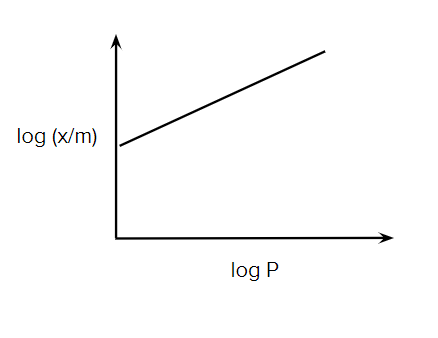a. 1/n (0 to 1)
b. 1/n (0.1 to 0.5)
c. log n
d. log (1/n)

According to Freundlich adsorption isotherm, log (x/m) = log K + 1/n log P.

So, the slope of the graph of log (x/m) vs log P is 1/n. Where, 1/n is from 0 to 1.

Question 19. The composition of gun metal is:
a. Cu, Zn, Sn
b. Al, Mg, Mn, Cu
c. Cu, Ni, Fe
d. Cu, Sn, Fe

The components of gun metal are:

Copper ~ 88%

Tin ~ 8%

Zinc ~ 4%

Majorly, copper

Question 20. Arrange Mg, Al, Si, P and S in the correct order of their ionisation potentials.

Answer: P > S > Si > Mg > Al

In the third period of the periodic table,

 Element Valence Shell Configuration Na 3s1 Mg 3s2 Al 3s23p1 Si 3s23p2 P 3s23p3 S 3s23p4

Ideally, from left to right, ionization enthalpy increases. But we see exceptions in (Mg, Al) & (P, S) where the element to the left, Mg and P, have higher ionization enthalpies compared to their respective immediate neighbors due to stable electronic configuration.

So, the order will be: P > S > Si > Mg > Al

Section B

Question 1. Cl2(g) ? 2Cl(g)

For the given reaction at equilibrium, moles of Cl2(g) is equal to the moles of Cl(g) and the equilibrium pressure is 1atm. If Kp of this reaction is x ×10–1, find x.

According to the question: Cl2 ? 2Cl [Ptotal = 1 atm].

Moles of Cl2 = Moles of Cl (at equilibrium).

Given: Kp = x ×10-1

nCl2 = nCl.

Therefore, PCl2 = PCl

Hence, PCl2 = PCl = 0.5 atm

kp= (PCl)2/ PCl2 = 0.5 = 5 x 10-1

So, x = 5

Question 2. S8 + bOH ? cS2- + sS2O2-3 + H2O. Find the value of c.

Let us look at the half reactions i.e. oxidation and reduction separately.

Oxidation:

S8 ? S2O2-3

S8 + 24OH ? 4S2O2-3 + 12H2O +16e ….(1)

Reduction:

S8 ? S2-

S8 + 16e ? 8S2- ….(2)

Adding both the reactions (1) and (2),

2S8 + 24OH ? 4S2O2-3 + 8S2- + 12H2O

Dividing the whole equation by 2,

S8 + 12OH ? 2S2O2-3 + 4S2- + 6H2O

So, c = 4

Question 3. Calculate the time taken in seconds for 40% completion of a first order reaction, if its rate constant is 3.3× 10-4 sec-1.

$$\begin{array}{l}t = \frac{1}{k}ln\frac{[A_{o}]}{[A]} = \frac{1\times 2.303}{3.33\times 10^{-4}}log\frac{}{} = \frac{1\times 2.303}{3.33\times 10^{-4}}\times 0.22\end{array}$$

= 30 x 103 x 0.506 = 1518 sec

Question 4. For a chemical reaction, Keq is 100 at 300K, the value of ?Go is –xR Joule at 1 atm pressure. Find the value of x. (Use ln 10 = 2.3)

Given: Keq= 100 at 300K and ?Go = –xR Joule

?Go = -RTln(Keq) = -2.303RTlog(Keq) = -1381.8R

Therefore, x = 1381.8 or 1382

Question 5. Cu2+ + NH3 ? [Cu(NH3)]2+ K1 = 104

[Cu(NH3)]2+ + NH3 ? [Cu(NH3)]2+ K2 = 1.58 × 103

[Cu(NH3)2]2+ + NH3 ? [Cu(NH3)3]2+ K3 = 5 × 102

[Cu(NH3)3]2+ + NH3 ? [Cu(NH3)4]2+ K4 = 102

If the dissociation constant of [Cu(NH3)4]2+ is X × 1012. Determine X.

Overall reaction constant (ß):

ß = K1 × K2 × K3 × K4

= 104 (1.58 ×103) × 5 × 102 × 102 = 7.9 × 1011

Cu2+ + 4NH3 ? [Cu(NH3)4]2+ ; ß = 7.9 × 1011

So, the dissociation constant (KDisso.) will be:

$$\begin{array}{l}\frac{1}{\beta } = \frac{1}{7.9 \times 10^{11}}\end{array}$$

KDisso = 1 / ß = 1.26 × 10-12

Hence, the value of X = 1.26

Question 6. 9.45g of CH2ClCOOH is dissolved in 500 mL of H2O solution and the depression in freezing point of the solution is 0.5°C. Find the percentage dissociation.

(Kf)H2O = 1.86K kg mole-1.

CH2Cl COOH CH2ClCOO + H+

Initial 1 0 0

Dissociated a a a

Left (1-a) a a

i = final moles / initial moles = 1 – a + a + a / 1 = 1 + a

?Tf = i x Kf × m

0.5 = (1 + a) × 1.86 × m

Molality (m) = nB / WA (kg) = WBMB x WA (kg) = 9.45 × 1000 / 94.5 × 500 = 0.2

Here, A is for solute and B is for solvent

WA=WH2O = 500g (Density of H2O = 1g/mL)

0.5 = (1+ a) ×1.86 × 0.2

a = 0.344

Percentage of dissociation = 34.4%

Question 7. What is the coordination number in Body Centered Cubic (BCC) arrangement of identical particles?

The easiest way is to look at the atom at the body center. It lies at the center of the body diagonal and touches the eight corner atoms. So, coordination number = 8.

Question 8. Among the following compounds, how many are amphoteric in nature?

Be(OH)2, BeO, Ba(OH)2, Sr(OH)2

The oxide and hydroxide of Be are amphoteric. Hence BeO and Be(OH)2 is amphoteric. Ba(OH)2 and Sr(OH)2 are basic.

Question 9. 4.5 g of a solute having molar mass of 90 g/mol is dissolved in water to make a 250 mL solution. Calculate the molarity of the solution.

WB (given weight of solute) = 4.5 g

MB (Molar mass of solute) = 90 g/mol

VS (Volume of solution) = 250 mL

= 250 / 1000 L = 1/4 L

Molarity (M) = nB / VS(L) = WB / MB.VS(L = 4.5 / (90 ×1/4) = 0.2 molar

Question 10. The mass of Li3+ is 8.33 times the mass of a proton. If Li3+ and proton are accelerated through the same potential difference, then the ratio of de Broglie’s wavelength of Li3+ to proton is x ×10–1. Find x

m (Li3+) = 8.33 × m p+ (given)

Debroglie’s wavelength (?) = h / p

KE = ½ mv2

Multiplying by m on both sides

m. KE = ½ mv2

2m. KE = (mv)2 = p2

P = v2m. KE

Also KE= q × V

So, P = 2mq.V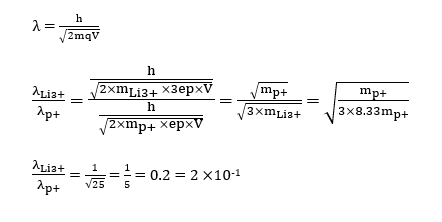Comparing with x × 10 -1 = 2 × 10 -1

x = 2

#### Video Solutions- February 2021 Question Papers